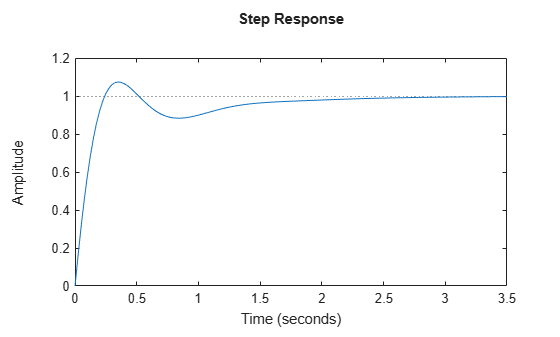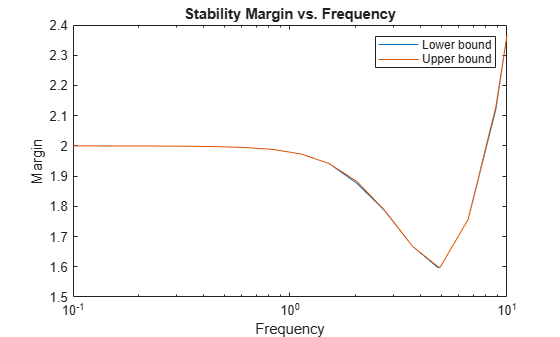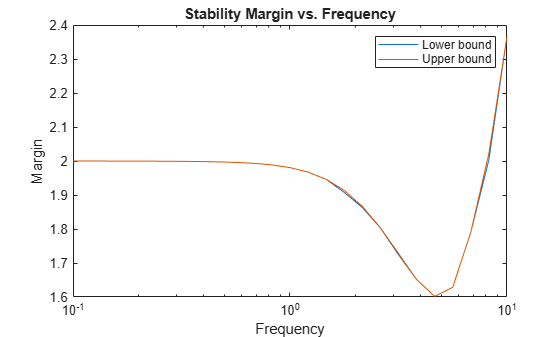# robstab

Robust stability of uncertain system

## Syntax

``````[stabmarg,wcu] = robstab(usys)``````
``````[stabmarg,wcu] = robstab(usys,w)``````
``````[stabmarg,wcu] = robstab(___,opts)``````
``````[stabmarg,wcu,info] = robstab(___)``````

## Description

example

``````[stabmarg,wcu] = robstab(usys)``` calculates the robust stability margin for an uncertain system. This stability margin is relative to the uncertainty level specified in `usys`. A robust stability margin greater than 1 means that the system is stable for all values of its modeled uncertainty. A robust stability margin less than 1 means that the system becomes unstable for some values of the uncertain elements within their specified ranges. For example, a margin of 0.5 implies the following: `usys` remains stable as long as the uncertain element values stay within 0.5 normalized units of their nominal values.There is a destabilizing perturbation of size 0.5 normalized units.The structure `stabmarg` contains upper and lower bounds on the actual stability margin and the critical frequency at which the stability margin is smallest. The structure `wcu` contains the destabilizing values of the uncertain elements.```

example

``````[stabmarg,wcu] = robstab(usys,w)``` restricts the robust stability margin computation to the frequencies specified by `w`. If `w` is a cell array of the form `{wmin,wmax}`, then `robstab` restricts the stability margin computation to the interval between `wmin` and `wmax`.If `w` is a vector of frequencies, then `robstab` computes the robust stability margin at the specified frequencies only.```

example

``````[stabmarg,wcu] = robstab(___,opts)``` specifies additional options for the computation. Use `robOptions` to create `opts`. You can use this syntax with any of the previous input-argument combinations.```

example

``````[stabmarg,wcu,info] = robstab(___)``` returns a structure with additional information about the stability margins and destabilizing perturbations. See `info` for details about this structure. You can use this syntax with any of the previous input-argument combinations.```

## Examples

collapse all

Consider a control system whose plant contains both parametric uncertainty and dynamic uncertainty. Create a model of the plant using uncertain elements.

```k = ureal('k',10,'Percent',40); delta = ultidyn('delta',[1 1]); G = tf(18,[1 1.8 k]) * (1 + 0.5*delta);```

Create a model of the controller, and build the closed-loop transfer function.

```C = pid(2.3,3,0.38,0.001); CL = feedback(G*C,1);```

A step response plot shows that the closed-loop system is nominally stable.

`step(CL.NominalValue)`Examine the robust stability of the closed-loop system.

```[stabmarg,wcu] = robstab(CL); stabmarg```
```stabmarg = struct with fields: LowerBound: 1.5960 UpperBound: 1.5993 CriticalFrequency: 4.8627 ```

The `LowerBound` and `UpperBound` fields of `stabmarg` show the robust stability margin of the closed-loop system is around 1.6. This result means that the system can withstand about 60% more uncertainty than is specified in the uncertain elements without going unstable.

You can use `uscale` to scale system uncertainty by the stability margin, to examine the system response for the full range of safe uncertainties. Scale the uncertainties in CL by the robust stability margin to create a system with the maximum tolerable amount of uncertainty.

```CLmaxunc = uscale(CL,stabmarg.UpperBound); CLmaxunc.Uncertainty.delta```
```ans = Uncertain LTI dynamics "delta" with 1 outputs, 1 inputs, and gain less than 1.6. ```
`CLmaxunc.Uncertainty.k`
```ans = Uncertain real parameter "k" with nominal value 10 and variability [-64,64]%. ```

The uncertain elements in `CLmaxunc` have ranges about 1.6 times the range of the original modeled uncertainty in `CL`.

The output `wcu` is a structure that contains the smallest perturbation to `k` and `delta` that make the system unstable. Confirm the instability by substituting these values into the closed-loop model and examining the pole locations.

```CLunst = usubs(CL,wcu); pole(CLunst)```
```ans = 8×1 complex 102 × -9.9314 + 0.0000i -0.1027 + 0.1009i -0.1027 - 0.1009i -0.0000 + 0.0486i -0.0000 - 0.0486i -0.0115 + 0.0000i -0.0216 + 0.0000i -0.0403 + 0.0000i ```

The resulting system has an undamped pair of complex poles with natural frequency 4.89, which renders it unstable. The `CriticalFrequency` field of `stabmarg` contains the same value, which is the frequency at which the `CL` is closest to instability.

Examine the relative sensitivity of the robust stability margin to the uncertain elements of the system. Consider a model of a control system containing uncertain elements.

```k = ureal('k',10,'Percent',40); delta = ultidyn('delta',[1 1]); G = tf(18,[1 1.8 k]) * (1 + 0.25*delta); C = pid(2.3,3,0.38,0.001); CL = feedback(G*C,1);```

Create an options set for `robstab` that enables the sensitivity calculation.

`opts = robOptions('Sensitivity','On');`

Calculate the robust stability margin, specifying the `info` output to access additional information about the calculation.

`[stabmarg,wcu,info] = robstab(CL,opts);`

Examine the `Sensitivity` field of `info`.

`info.Sensitivity`
```ans = struct with fields: delta: 80 k: 20 ```

The values in this field indicate how much a change in the normalized perturbation on each element affects the stability margin. For example, the sensitivity for `k` is 21. This value means that a given change `dk` in the normalized uncertainty range of `k` causes a change of about 21% percent of that, or `0.21*dk`, in the stability margin. The margin in this case is much more sensitive to `delta`, for which the margin changes by about 81% of the change in the normalized uncertainty range.

Consider a model of a control system containing uncertain elements.

```k = ureal('k',10,'Percent',40); delta = ultidyn('delta',[1 1]); G = tf(18,[1 1.8 k]) * (1 + 0.5*delta); C = pid(2.3,3,0.38,0.001); CL = feedback(G*C,1);```

By default, `robstab` computes only the weakest stability margin over all frequencies. To see how the stability margin varies with frequency, use the `'VaryFrequency'` option of `robOptions`. For example, compute the stability margin of the system at frequency points between 0.1 and 10 rad/s.

```opts = robOptions('VaryFrequency','on'); [stabmarg,wcu,info] = robstab(CL,{0.1,10},opts); info```
```info = struct with fields: Model: 1 Frequency: [19x1 double] Bounds: [19x2 double] WorstPerturbation: [19x1 struct] Sensitivity: [1x1 struct] ```

`robstab` returns the vector of frequencies in the `info` output, in the `Frequencies` field. `info.Bounds` contains the upper and lower bounds on the stability margin at each frequency. Use these values to plot the frequency dependence of the stability margin.

```semilogx(info.Frequency,info.Bounds) title('Stability Margin vs. Frequency') ylabel('Margin') xlabel('Frequency') legend('Lower bound','Upper bound')```When you use the `'VaryFrequency'` option, `robstab` chooses frequency points automatically. The frequencies it selects are guaranteed to include the frequency at which the stability margin is weakest (within the specified range). Display the returned frequency values to confirm that they include the critical frequency.

`info.Frequency`
```ans = 19×1 0.1000 0.1061 0.1425 0.1914 0.2572 0.3455 0.4642 0.6236 0.8377 1.1253 ⋮ ```
`stabmarg.CriticalFrequency`
```ans = 4.8280 ```

Alternatively, instead of using `'VaryFrequency'`, you can specify particular frequencies at which to compute the robust stability margins. `info.Bounds` contains the margins at all specified frequencies. However, these results are not guaranteed to include the weakest margin, which might fall between specified frequency points.

```w = logspace(-1,1,25); [stabmarg,wcu,info] = robstab(CL,w); semilogx(w,info.Bounds) title('Stability Margin vs. Frequency') ylabel('Margin') xlabel('Frequency') legend('Lower bound','Upper bound')```## Input Arguments

collapse all

Dynamic system with uncertainty, specified as a `uss`, `ufrd`, `genss`, or `genfrd` model that contains uncertain elements. For `genss` or `genfrd` models, `robstab` uses the current value of any tunable blocks and folds them into the known (not uncertain) part of the model.

For frequency-response models, `ufrd` or `genfrd`, `robstab` assumes that the system is nominally stable.

`usys` can also be an array of uncertain models. In that case, `robstab` returns the smallest margin across all models in the array, and the `info` output contains the index of the corresponding model.

Frequencies at which to compute robust stability margins, specified as the cell array `{wmin,wmax}` or as a vector of frequency values.

• If `w` is a cell array of the form `{wmin,wmax}`, then the function computes the margins at frequencies ranging between `wmin` and `wmax`.

• If `w` is a vector of frequencies, then the function computes the margins at each specified frequency. For example, use `logspace` to generate a row vector with logarithmically spaced frequency values.

For `uss` and `genss` models, when `w` is a vector, `robstab(usys,w)` is equivalent to `robstab(ufrd(usys,w))`. Therefore, `usys` must be nominally stable.

Specify frequencies in units of rad/`TimeUnit`, where `TimeUnit` is the `TimeUnit` property of the model.

Options for computation of robust stability margins, specified as an object you create with `robOptions`. The available options include settings that let you:

• Extract frequency-dependent stability margins.

• Examine the sensitivity of the margins to each uncertain element.

• Improve the results of the stability-margin calculation by setting certain options for the underlying `mussv` calculation. In particular, setting the option `'MussvOptions'` to `'mN'` can reduce the gap between the lower bound and upper bound. `N` is the number of restarts.

For more information about all available options, see `robOptions`.

Example: `robOptions('Sensitivity','on','MussvOptions','m3')`

## Output Arguments

collapse all

Robust stability margin and critical frequency, returned as a structure containing the following fields:

FieldDescription

`LowerBound`

Lower bound on the actual robust stability margin of the model, returned as a scalar value. The exact stability margin is guaranteed to be no smaller than `LowerBound`. In other words, for all modeled uncertainty with normalized magnitude up to `LowerBound`, the system is guaranteed stable.

`UpperBound`

Upper bound on the actual robust stability margin of the model, returned as a scalar value. The exact stability margin is guaranteed to be no larger than `UpperBound`. In other words, there exist some uncertain-element values associated with this magnitude that cause instability. `robstab` returns one such instance in `wcu`.

`CriticalFrequency`

Frequency at which the stability margin is the smallest, in rad/`TimeUnit`, where `TimeUnit` is the `TimeUnit` property of `usys`.

A robust stability margin greater than 1 means that `usys` is stable for all values of its modeled uncertainty. A robust stability margin less than 1 implies that `usys` becomes unstable for some values of its uncertain elements within their specified ranges. For example, a margin of 0.5 implies the following:

• `usys` remains stable as long as the uncertain element values stay within 0.5 normalized units of their nominal values.

• There is a destabilizing perturbation of size 0.5 normalized units.

Use `uscale` to scale the amount of uncertainty in `usys` by the stability margin to examine the actual tolerable ranges of uncertainty.

If the nominal value of `usys` is unstable, the stability margin is 0. If `usys` is a `ufrd` or `genfrd` model, `robstab` assumes it is nominally stable.

If `usys` is an array of uncertain models, `stabmarg` contains the smallest margin across all models in the array. In that case, the `info` output contains the index of the corresponding model in its `Model` field.

Smallest perturbations of uncertain elements that cause instability in `usys`, returned as a structure whose fields are the names of the uncertain elements of `usys`. Each field contains the actual destabilizing value for each uncertain element of `usys`. For example, if `usys` includes an uncertain matrix `M` and SISO uncertain dynamics `delta`, then `wcu.M` is a numeric matrix and `wcu.delta` is a SISO state-space model.

Use `usubs(usys,wcu)` to substitute these values for the uncertain elements in `usys`, to obtain the unstable dynamic system that deviates the least from the nominal system. Use `actual2normalized` to convert these actual uncertainty values to the normalized units in which the stability margin is expressed.

For `ureal` parameters in `usys` whose range is not centered around the nominal value, `robstab` makes the following adjustments for the purposes of its analysis:

• When the worst perturbation (the smallest destabilizing perturbation) lies outside the range of validity of the actual-to-normalized transformation (see `getLimits`), then `robstab` sets the corresponding entry of `wcu` to the nearest valid value. In other words, if `actpert` is the worst perturbation in actual units, `robgain` sets `wcu` to the nearest value inside the interval `ActLims` returned by `getLimits`.

• When there is no destabilizing perturbation, then `robstab` sets the corresponding entry of `wcu` to the nominal value of the `ureal` parameter.

Additional information about the robust stability margins, returned as a structure with the following fields:

FieldDescription

`Model`

Index of the model that has the weakest stability margin, when `usys` is an array of models.

`Frequency`

Frequency points at which `robstab` returns the robust stability margin, returned as a vector.

• If the `'VaryFrequency'` option of `robOptions` is `'off'`, then `info.Frequency` is the critical frequency, the frequency at which the smallest margin occurs. If the smallest lower bound and the smallest upper bound on the stability margin occur at different frequencies, then `info.Frequency` is a vector containing these two frequencies.

• If the `'VaryFrequency'` option of `robOptions` is `'on'`, then `info.Frequency` contains the frequencies selected by `robstab`. These frequencies are guaranteed to include the frequency at which the stability margin is smallest.

• If you specify a vector of frequencies `w` at which to compute the stability margins, then ```info.Frequency = w```. When you specify a frequency vector, these frequencies are not guaranteed to include the frequency at which the stability margin is smallest.

The `'VaryFrequency'` option is meaningful only for `uss` and `genss` models. `robstab` ignores the option for `ufrd` and `genfrd` models.

`Bounds`

Lower and upper bounds on the actual robust stability margin of the model, returned as an array. `info.Bounds(:,1)` contains the lower bound at each corresponding frequency in `info.Frequency`, and `info.Bounds(:,2)` contains the corresponding upper bounds.

`WorstPerturbation`

Smallest destabilizing perturbations at each frequency point in `info.Frequency`, returned as a structure array. The fields of `info.WorstPerturbation` are the names of the uncertain elements in `usys`, and each field contains the destabilizing value of the corresponding element at each frequency. For example, if `usys` includes an uncertain parameter `p` and SISO uncertain dynamics `delta`, then `info.WorstPerturbation.p` is a collection of numeric values and `info.WorstPerturbation.delta` is a collection of SISO state-space models.

`Sensitivity`

Sensitivity of the stability margin to each uncertain element, returned as a structure when the `'Sensitivity'` option of `robOptions` is `'on'`. The fields of `info.Sensitivity` are the names of the uncertain elements in `usys`. Each field contains a percentage that measures how much the uncertainty in the corresponding element affects the stability margin. For example, if `info.Sensitivity.p` is 50, then a given fractional change in the uncertainty range of `p` causes half as much fractional change in the stability margin.

If the `'Sensitivity'` option of `robOptions` is off (the default setting), then `info.Sensitivity` is `NaN`.

## Algorithms

Computing the robustness margin at a particular frequency is equivalent to computing the structured singular value, μ, for some appropriate block structure (μ-analysis).

For `uss` and `genss` models, `robstab(usys)` and `robstab(usys,{wmin,wmax})` use an algorithm that finds the smallest margin across frequency. This algorithm does not rely on frequency gridding and is not adversely affected by discontinuities of the μ structured singular value. See Getting Reliable Estimates of Robustness Margins for more information.

For `ufrd` and `genfrd` models, `robstab` computes the μ lower and upper bounds at each frequency point. This computation offers no guarantee between frequency points and can be inaccurate if there are discontinuities or sharp peaks in μ. The syntax `robstab(uss,w)`, where `w` is a vector of frequency points, is the same as `robstab(ufrd(uss,w))` and also relies on frequency gridding to compute the margin.

In general, the algorithm for state-space models is faster and safer than the frequency-gridding approach. In some cases, however, the state-space algorithm requires many μ calculations. In those cases, specifying a frequency grid as a vector `w` can be faster, provided that the robustness margin varies smoothly with frequency. Such smooth variation is typical for systems with dynamic uncertainty.